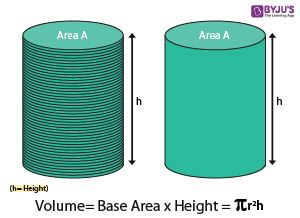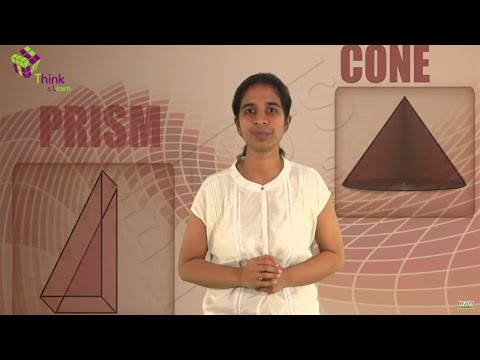# Volume of a Cylinder

The volume of a cylinder is the density of the cylinder which signifies the amount of material it can carry or how much amount of any material can be immersed in it. Cylinder’s volume is given by the formula, πr2h, where r is the radius of the circular base and h is the height of the cylinder. The material could be a liquid quantity or any substance which can be filled in the cylinder uniformly. Check volume of shapes here.

Volume of cylinder has been explained in this article briefly along with solved examples for better understanding. In Mathematics, geometry is an important branch where we learn the shapes and their properties. Volume and surface area are the two important properties of any 3d shape.

## Definition

The cylinder is a three-dimensional shape having a circular base. A cylinder can be seen as a set of circular disks that are stacked on one another. Now, think of a scenario where we need to calculate the amount of sugar that can be accommodated in a cylindrical box.

In other words, we mean to calculate the capacity or volume of this box. The capacity of a cylindrical box is basically equal to the volume of the cylinder involved. Thus, the volume of a three-dimensional shape is equal to the amount of space occupied by that shape.

## Volume of a Cylinder Formula

A cylinder can be seen as a collection of multiple congruent disks, stacked one above the other. In order to calculate the space occupied by a cylinder, we calculate the space occupied by each disk and then add them up. Thus, the volume of the cylinder can be given by the product of the area of base and height.For any cylinder with base radius ‘r’, and height ‘h’, the volume will be base times the height.

Therefore, the cylinder’s volume of base radius ‘r’, and height ‘h’ = (area of base) × height of the cylinder

Since the  base is the circle, it can be written as

Volume =  πr× h

Therefore, the volume of a cylinder = πr2h cubic units.### Volume of Hollow Cylinder

In case of hollow cylinder, we measure two radius, one for inner circle and one for outer circle formed by the base of hollow cylinder. Suppose, r1 and r2 are the two radii of the given hollow cylinder with ‘h’ as the height, then the volume of this cylinder can be written as;

• V =  πh(r12 – r22)

### Surface Area of Cylinder

The amount of square units required to cover the surface of the cylinder is the surface area of the cylinder. The formula for the surface area of the cylinder is equal to the total surface area of the bases of the cylinder and surface area of its sides.

• A = 2πr2 + 2πrh

#### Volume of Cylinder in Litres

When we find the volume of the cylinder in cubic centimetres, we can convert the value in litres by knowing the below conversion, i.e.,

1 Litre = 1000 cubic cm or cm3
For example: If a cylindrical tube has a volume of 12 litres, then we can write the volume of the tube as 12 × 1000 cm3 = 12,000 cm3

### Examples

Question 1: Calculate the volume of a given cylinder having height 20 cm and base radius of 14 cm. (Take pi = 22/7)

Solution:

Given:

Height  = 20 cm

we know that;

Volume, V = πr2h  cubic units

V=(22/7) × 14  × 14  × 20

V= 12320 cm3

Therefore, the volume of a cylinder = 12320 cm3

Question 2: Calculate the radius of the base of a cylindrical container of volume 440 cm3. Height of the cylindrical container is 35 cm. (Take pi = 22/7)

Solution:

Given:

Volume = 440 cm3

Height = 35 cm

We know from the formula of cylinder;

Volume, V = πr2h  cubic units

So, 440 = (22/7) × r2 × 35

r= (440 × 7)/(22 × 35) = 3080/770 = 4

Therefore, r = 2 cm

Therefore, the radius of a cylinder = 2 cm.